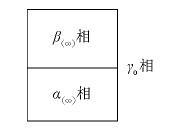## Derivation of Kelvin Equation for the Phase Equilibrium between the Curved Interface Based on Gibbs Thermodynamics,, , , ,

 基金资助: 国家自然科学基金.  51204001国家自然科学基金.  51404001

 Fund supported: 国家自然科学基金.  51204001国家自然科学基金.  51404001Abstract

In this paper, two interface thermodynamics methods, the Guggenheim method and the Gibbs method, are introduced.The thermodynamic criterion of the two-phase equilibrium is established using Gibbs interface thermodynamics, and a new method for derivation of Kelvin equation is put forward based on the thermodynamic criterion of the two-phase equilibrium.

Keywords： Kelvin Equation ; Guggenheim interface thermodynamics ; Gibbs interface thermodynamics

XIAO Sai-Jun, ZHANG Jun, YIN Zhen-Xing, WANG Zhen, LIU Jian. Derivation of Kelvin Equation for the Phase Equilibrium between the Curved Interface Based on Gibbs Thermodynamics. University Chemistry[J], 2016, 31(1): 59-63 doi:10.3866/pku.DXHX20160159

## 1 建立界面热力学的两种方法

### 图1$\text{d}G\text{=d}G\alpha \text{+d}G\beta$

$\text{d}{{G}_{\alpha }}=-{{S}_{\alpha }}\text{d}T+{{V}_{\alpha }}\text{d}p+\sum\limits_{i}{{{\mu }_{\alpha }}}\text{d}{{n}_{\alpha }}$

$\text{d}{{G}_{\gamma }}=-{{S}_{\gamma }}\text{d}T+{{V}_{\gamma }}\text{d}p+\sum\limits_{i}{{{\mu }_{\gamma }}}\text{d}{{n}_{\gamma }}$

$\text{d}{{G}_{\beta }}=-{{S}_{\beta }}\text{d}T+{{V}_{\beta }}\text{d}p+\sum\limits_{i}{{{\mu }_{\beta }}}\text{d}{{n}_{\beta }}$

### 图2$\text{d}{{G}_{\text{表面}}}\text{=}{{\left(\frac{\partial {{G}_{\text{总}}}}{\partial A} \right)}_{T,p,{{n}_{i}}}}\text{d}{{A}_{\text{s}}}\text{=}\sigma \text{d}{{A}_{\text{s}}}$

$\text{d}G\text{=d}{{G}_{\alpha \left(\infty \right)}}\text{+d}{{G}_{\beta \left(\infty \right)}}\text{+d}{{G}_{\text{表面}}}$

$\text{d}G\text{=d}{{G}_{\alpha \left(\infty \right)}}\text{+d}{{G}_{\beta \left(\infty \right)}}\text{+d}{{G}_{\text{表面}}}\text{=d}{{G}_{\alpha \left(\infty \right)}}\text{+d}{{G}_{\beta \left(\infty \right)}}\text{+}\sigma \text{d}{{A}_{\text{s}}}$

### 2 有界面相时两相相平衡的热力学判据

${{\mu }_{i}}^{\text{A}}(T,{{p}^{A}})\text{=}{{\mu }_{i}}^{\text{B}}(T,{{p}^{B}})$

$\text{d}G\text{=d}{{G}_{\text{A}}}\text{+d}{{G}_{\text{B}}}\text{+}\sigma \text{d}{{A}_{\text{s}}}\text{=}-{{\mu }_{i}}^{\text{A}}\text{d}{{n}_{i}}\text{+}{{\mu }_{i}}^{\text{B}}\text{d}{{n}_{i}}\text{+}\sigma \text{d}{{A}_{\text{s}}}=0$

### 2.1 界面相为平面

${{\mu }_{i}}^{\text{A}}\text{=}{{\mu }_{i}}^{\text{B}}$

### 2.2 界面相为弯曲面

$-{{\mu }_{i}}^{\text{A}}\text{d}{{n}_{i}}\text{+}{{\mu }_{i}}^{\text{B}}\text{d}{{n}_{i}}\text{+}\sigma \text{d}{{A}_{\text{s}}}=或{{\mu }_{i}}^{\text{B}}\text{d}{{n}_{i}}\text{+}\sigma \text{d}{{A}_{\text{s}}}={{\mu }_{i}}^{\text{A}}\text{d}{{n}_{i}}$

### 3.1 开尔文方程推导新方法

$-{{\mu }_{气}}\text{d}n\text{+}{{\mu }_{液}}\text{d}n\text{+}\sigma \text{d}{{A}_{\text{s}}}=0$

$\sigma(\frac{\text{d}{{A}_{\text{s}}}}{\text{d}n})={{\mu }_{气}}-{{\mu }_{液}}$

$\sigma(\frac{\text{d}{{A}_{\text{s}}}}{\text{d}n})={{\mu }_{气}}-{{\mu }_{液}}\text{=}\left(\mu _{气}^{\theta }\ \text{+}RT\ln \frac{p_{2}^{*}}{{{p}^{\theta }}} \right)-\left(\mu _{气}^{\theta }\ \text{+}RT\ln \frac{p_{1}^{*}}{{{p}^{\theta }}} \right)\text{=}RT\ln \frac{p_{2}^{*}}{p_{1}^{*}}$

$\frac{2\sigma M}{r\rho }\text{=}RT\ln \frac{p_{2}^{*}}{p_{1}^{*}}$

### 图3## 4 结论

①依据吉布斯界面热力学，当界面相为平面时，两相平衡的热力学判据为${\mu _i}^{\rm{A}}{\rm{=}}{\mu _i}^{\rm{B}}$；当界面相为曲面时，两相平衡的热力学判据为 ${\mu _i}^{\rm{B}}{\rm{d}}{n_i}{\rm{+}}\sigma {\rm{d}}{A_{\rm{s}}}={\mu _i}^{\rm{A}}{\rm{d}}{n_i}$

②基于吉布斯界面热力学理论及其推演得到的存在界面相时相平衡热力学判据可以推导出开尔文方程。该推导过程基于相平衡的热力学第二定律，物理模型清晰，推导过程简洁，热力学意义明确。

## 参考文献 原文顺序 文献年度倒序 文中引用次数倒序 被引期刊影响因子

Berg J.C. An Introduction to Interfaces of Colloids: The Bridge to Nanoscience Singapore: World Scientific Publishing Co.Pte.Ltd., 2009,

/

 〈〉